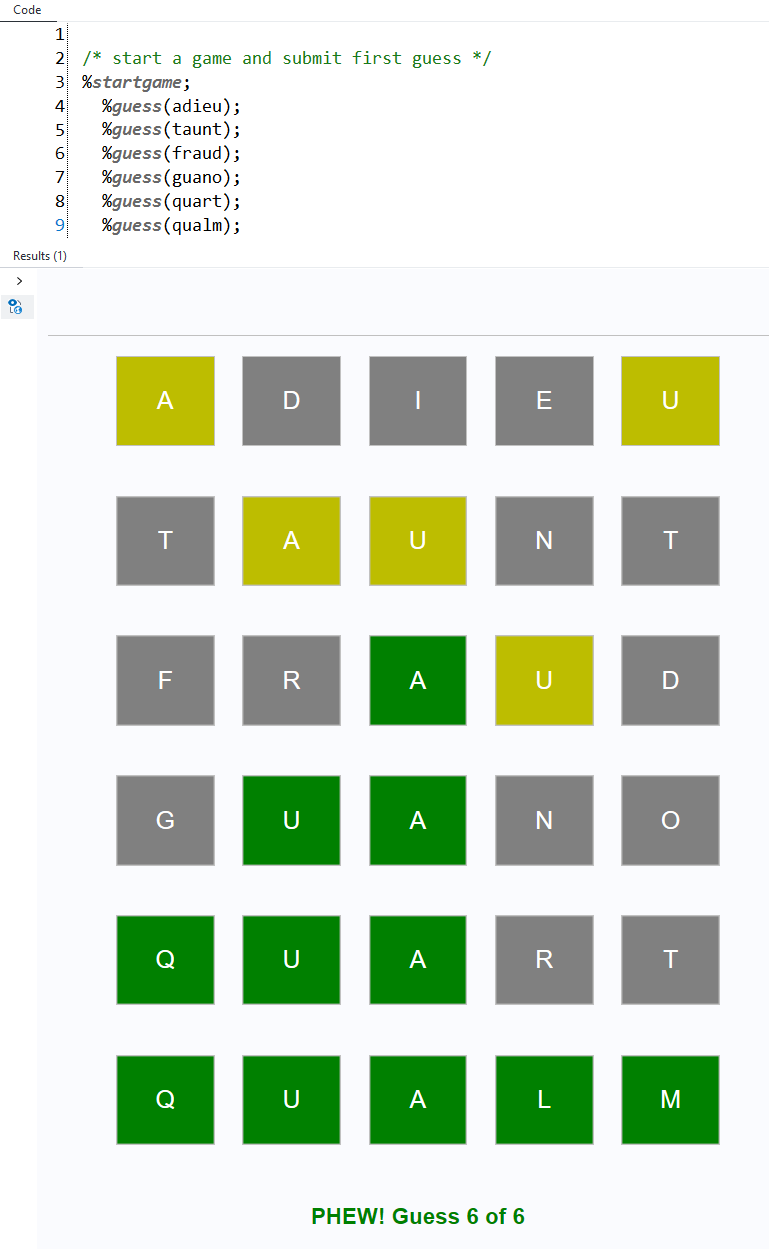Instantly share code, notes, and snippets.

# huytd/wordle.md

Last active October 9, 2023 02:23
Star You must be signed in to star a gist
Wordle in less than 50 lines of Bash
This file contains bidirectional Unicode text that may be interpreted or compiled differently than what appears below. To review, open the file in an editor that reveals hidden Unicode characters. Learn more about bidirectional Unicode characters
 words=(\$(grep '^\w\w\w\w\w\$' /usr/share/dict/words | tr '[a-z]' '[A-Z]')) actual=\${words[\$[\$RANDOM % \${#words[@]}]]} end=false guess_count=0 max_guess=6 if [[ \$1 == "unlimit" ]]; then max_guess=999999 fi while [[ \$end != true ]]; do guess_count=\$(( \$guess_count + 1 )) if [[ \$guess_count -le \$max_guess ]]; then echo "Enter your guess (\$guess_count / \$max_guess):" read guess guess=\$(echo \$guess | tr '[a-z]' '[A-Z]') if [[ " \${words[*]} " =~ " \$guess " ]]; then output="" remaining="" if [[ \$actual == \$guess ]]; then echo "You guessed right!" for ((i = 0; i < \${#actual}; i++)); do output+="\033[30;102m \${guess:\$i:1} \033[0m" done printf "\$output\n" end=true else for ((i = 0; i < \${#actual}; i++)); do if [[ "\${actual:\$i:1}" != "\${guess:\$i:1}" ]]; then remaining+=\${actual:\$i:1} fi done for ((i = 0; i < \${#actual}; i++)); do if [[ "\${actual:\$i:1}" != "\${guess:\$i:1}" ]]; then if [[ "\$remaining" == *"\${guess:\$i:1}"* ]]; then output+="\033[30;103m \${guess:\$i:1} \033[0m" remaining=\${remaining/"\${guess:\$i:1}"/} else output+="\033[30;107m \${guess:\$i:1} \033[0m" fi else output+="\033[30;102m \${guess:\$i:1} \033[0m" fi done printf "\$output\n" fi else echo "Please enter a valid word with 5 letters!"; guess_count=\$(( \$guess_count - 1 )) fi else echo "You lose! The word is:" echo \$actual end=true fi done

### cjdinger commented Mar 17, 2022

I wrote a SAS version and placed it here sascommunities/wordle-sas. Uses the word lists from cfreshman (thanks) and arrays to check guesses.